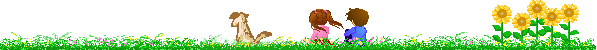## การใช้งาน Built in tuple ในภาษา python (function (d, s, id) { var js, fjs = d.getElementsByTagName(s); if (d.getElementById(id)) return; js = d.createElement(s); js.id = id; js.src = "//connect.facebook.net/en_EN/sdk.js#xfbml=1&version=v2.3&appId="; fjs.parentNode.insertBefore(js, fjs); }(document, 'script', 'facebook-jssdk'));

แชร์ความรู้ภาษา Python ไพทอน การเขียนโปรแกรมภาษาไพทอน

Moderators: mindphp, ผู้ดูแลกระดาน

Four
PHP Super Hero MemberPosts: 813
Joined: 08/01/2018 9:55 am

### การใช้งาน Built in tuple ในภาษา python

len(tuple) จำนวน element ทั้งหมดใน tuple

Code: Select all

``````test = ("python", "mindphp", "1234")
test2 = ("python", "mindphp")
print(len(test))
print(len(test2))``````
max(tuple) element ที่มีค่ามากที่สุด

Code: Select all

``````test3 = ("python", "mindphp", "tuple")
test4 = (123, 456, 789)
print(max(test3))
print(max(test4))``````
ผลรันI am slow walker, but I never walk back. (Abraham Lincoln)
Jom07
PHP Super Hero MemberPosts: 514
Joined: 08/01/2018 9:56 am

### Re: การใช้งาน Built in tuple ในภาษา python

min(tuple) element ที่มีค่ามากที่สุด

Code: Select all

``````x = ('name12', 'name123', 'name1234')
y = (1000, 2000, 3000)

print(min(x))
print(min(y))``````
ผลรันtuple(sqe)แปลง sequence (List ) ให้เป็น Tuple

Code: Select all

``````x = {'name12', 'name123', 'name1234'}
y =tuple(x)
print(y)
``````
ผลรัน